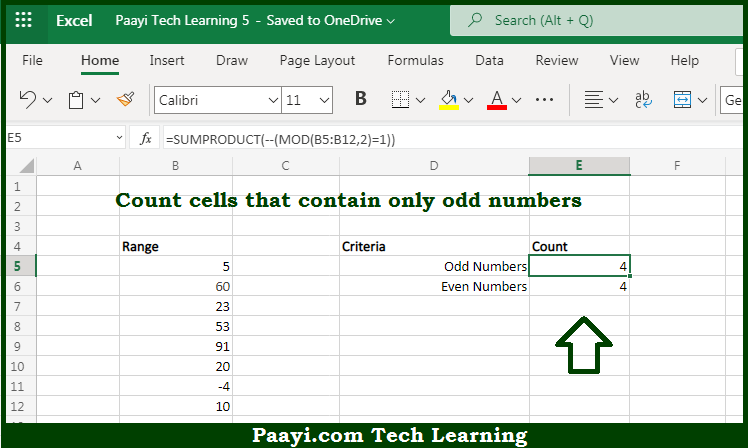# Learn How to Count Cells that Contain Odd Numbers in Microsoft Excel

Written by | 0 Comments | 470 Views

In this article, you will learn how to COUNT various things in Microsoft Excel using a single or combination of functions and other purposes. You will also get to know how to count cells that contain odd numbers and see the generic formula.

Count Cells that Contain Odd Numbers in Microsoft Excel

The main purpose of this formula is to count the number of cells that contain odd numbers. Here we will learn how to count cells containing the odd numeric values in Microsoft Excel.  That implies, with the help of a formula based on the  SUMPRODUCT function you can able to count the number of cells that contain only odd numbers. The generic form of the formula provided below "rng" represents a range of cells. So, with the help of this formula, you can able to count the number of cells that contain odd numbers.

General Formula to COUNT Cells that Contain Odd Numbers

=COUNT(rng)

The Explanation for the COUNT Cells that Contain Odd NumbersSo we know that with the help of the given formula above you can able to count the number of cells that contain odd numbers. Here we will learn how to count cells containing the odd numeric values in Microsoft Excel. As we know that, the SUMPRODUCT function functions directly with arrays. It should be noted that you can do one thing quite easily with the SUMPRODUCT function is performing a test on an array using one or more criteria, and count the results. In the example provided here, we are running a test for an odd number, that uses the MOD function. So, with the help of this formula, you can able to count cells that contain odd numbers.